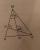Is right triangle

Decide if the triangle XYZ is rectangular:
x = 4 m, y = 6 m, z = 4 m

Result

j =  0

Solution:Leave us a comment of example and its solution (i.e. if it is still somewhat unclear...):Be the first to comment!To solve this example are needed these knowledge from mathematics:

Pythagorean theorem is the base for the right triangle calculator. See also our trigonometric triangle calculator.

Next similar examples:

1. Right trianglesHow many right triangles we can construct from line segments 3,4,5,6,8,10,12,13,15,17 cm long? (Do not forget to the triangle inequality).The double ladder is 8.5m long. It is built so that its lower ends are 3.5 meters apart. How high does the upper end of the ladder reach?The double ladder shoulders should be 3 meters long. What height will the upper top of the ladder reach if the lower ends are 1.8 meters apart?
4. Oil rigOil drilling rig is 23 meters height and fix the ropes which ends are 7 meters away from the foot of the tower. How long are these ropes?
5. StairwayStairway has 20 steps. Each step has a length of 22 cm and a height of 15 cm. Calculate the length of the handrail of staircases if on the top and bottom exceeds 10 cm.
6. A truckA truck departs from a distribution center. From there, it goes 20km west, 30km north and 10km west and reaches a shop. How can the truck reach back to the distribution center from the shop (what is the shortest path)?
7. Broken treeThe tree is broken at 4 meters above the ground and the top of the tree touches the ground at a distance of 5 from the trunk. Calculate the original height of the tree.
8. WindbreakA tree at a height of 3 meters broke in the windbreak. Its peak fell 4.5 m from the tree. How tall was the tree?
9. Broken treeThe tree was 35 meters high. The tree broke at a height of 10 m above the ground. Top but does not fall off it refuted on the ground. How far from the base of the tree lay its peak?
10. Cone 15The radius of the base of a right circular cone is 14 inches and it's height 18 inches. What is the slant height?
11. Common chordTwo circles with radius 17 cm and 20 cm are intersect at two points. Its common chord is long 27 cm. What is the distance of the centers of these circles?
12. Chord circleThe circle to the (S, r = 8 cm) are different points A, B connected segment /AB/ = 12 cm. AB mark the middle of S'. Calculate |SS'|. Make the sketch.
13. SatinSanusha buys a piece of satin 2.4 m wide. The diagonal length of the fabric is 4m. What is the length of the piece of satin?
14. Spruce heightHow tall was spruce that was cut at an altitude of 8m above the ground and the top landed at a distance of 15m from the heel of the tree?The ladder has a length 3.5 meters. He is leaning against the wall so that his bottom end is 2 meters away from the wall. Determine the height of the ladder.The line OH is the height of the triangle DOM, line MN is the bisector of angle DMO. obtuse angle between the lines MN and OH is four times larger than the angle DMN. What size is the angle DMO? (see attached image)It is true that the middle traverse bisects the triangle?